##### Page tree

MagicDraw 2021x Refresh1 Documentation

Operation nameDescriptionExample
Date
Timestamp

The operation returns current system time in milliseconds as a string type value. It takes no parameters.

Result = “1392798008000”
FormatDate

The operation converts a date and time given in milliseconds to a human readable format.

The operation takes two parameters:

• Date – a date and time in milliseconds that should be converted to a human readable format. It must be a string type value and can be the result of a Timestamp operation.
• Format – a date and time format for the conversion. It must be a string type value. For the date and time formats, refer to the SimpleDateFormat page.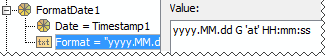The result of this operation is a string type value.

Date = “1392798008000”

Format = “yyyy.MM.dd G 'at'
HH:mm:ss z”

10:20:08 EET”

ParseDate

The operation converts a date and time in a human readable format to milliseconds. In other words, the operation reverses the result of the FormatDate operation (it returns the value that can be the Date parameter for a FormatDate operation).

The operation takes two parameters:

• Format – a current format of the date and time that should be converted to milliseconds. It must be a string type value.
• Formatted Date – a date and time that should be converted to milliseconds. It must be a string type value.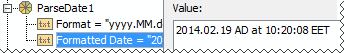The result of this operation is a string type value.

Format = “yyyy.MM.dd G 'at'
HH:mm:ss z”

Formatted Date =
EET”

Result = “1392798008000”

Collection
TypeTest

The operation tests, whether the type of an element matches a given type or stereotype. If the types matches, it returns true, and if they not – false.

You can also use this operation to check, if an element is an instance of a given classifier.

The operation takes three parameters:

• Element – a model element, whose type you need to test.
• Type – a type, stereotype, or classifier for testing the element.
• Include Subtypestrue, if the inherited types, stereotypes, or classifiers of the selected Type parameter value should be included in the test; false, if not.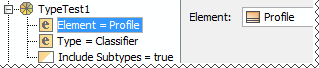The TypeTest operation can be used in the Filter operation as the condition. For this, click the Use as Condition of a new Filter operation button on the Type Test operation specification panel.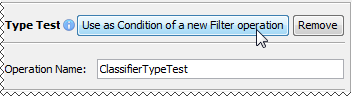Element = class Profile
Type = Class
Include Subtypes = false

Result = true

The element type matches the given type.

Element = class Profile
Type = Classifier
Include Subtypes = true

Result = true

Though the element type does not match the given type, it is its subtype, and in this case subtypes are included in the test.

Element = class Profile
Type = Classifier
Include Subtypes = false

Result = false

Thought the element type is a subtype of the given type, in this case subtypes are not included in the test.

Filter

The operation analyses given collection of elements (one by one) and returns only those elements that meet the specified filter criteria.

The operation takes two parameters:

• Input Collection – an arbitrary collection of elements of arbitrary types.
• Predicate – an operation determining filter criteria. Keep in mind that this operation must have exactly one input parameter and return a result of boolean type! Otherwise, the Filter operation fails and returns no value. Hence, if you need to use the script operation with many parameters as the filter criteria, you must wrap it inside the nested operation (the TypeTest operation or any other built-in operation is wrapped automatically).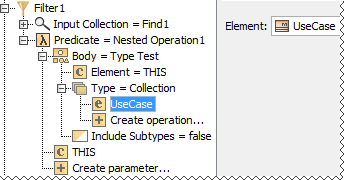The result of this operation is collection of elements as well.

Input Collection =

use case Log out
actor User

Predicate = TypeTest:

Element = Input Collection
Type = UseCase
Include Subtypes = false

Result Collection =

use case Log out

The operation finds the needed slot of the given instance and returns one or more values of that slot.

The operation takes two parameters:

• Instance – an instance specification (instance), whose slot value you need to access.
• Navigate Property – a property, whose slot you need to find.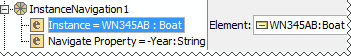The result type of this operation matches the type of the property specified as the Navigate Property parameter.

Instance = WN345AB
Navigate Property = Year

Result = “1999”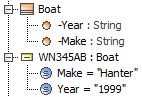First

The operation finds the first element in a sequence from a specified scope.

The operation consist of:

• Input - objects list.

Contains

The operation verifies specified object in given collection and returns Boolean value true if sets contains object, otherwise operation returns value false.

The operation consist of:

• Input - operation will verify this collections.
• Obj - sets will be verified according to this object.
Input =

IsEmpty

The operation determine whether collection is empty, if it is empty, operation returns Boolean value true, otherwise operation returns value false.

The operation consist of:

• Input - specified object (for example array, set, list).

Size

The operation returns the number of elements in collection.

The operation consist of:

• Input - an object or collection (for example array, set, list, string).

Intersect

The operation returns the data common to both collections, with no repetitions and in sorted order.

The operation consist of:

• Collection1 and Collection2 - collections which will be intersected.

C1 = [7 0 5], C2 = [7 1 5],

returns C = [5 7];

Concat

The operation joins two lists. The List1 and List2 are joined end-to-end. Keeps the order, allows duplicates.

The operation consist of:

• List1 and List2 - lists which will be concatenated.

Get

The operation returns the element at the specified position in collection.

The operation consist of:

• Input - specified collection.
• Index - index of the element to return (integer type).

Map

The operation maps objects from collection to collection of other objects and returns a collection of results in the same order.

The operation consist of:

• Input - collections with objects to map.
• MapOperation - given function, this function will be applied to each input element.

Input = [1 2 3 4 5],

applies operation map with function (Input + 1)

returns = [2 3 4 5 6]

MapFlat

The operation maps objects from collection to collection of other objects. Flattens mapping result if it is a collection.

The operation consist of:

• Input - collections with objects to map.
• MapOperation - given function, this function will be applied to each input element.

Input = { {1,2}, {3,4}, {5,6} }

applies operation MapFlat

returns = {1,2,3,4,5,6}

Reduce

The operation reduce collection using given operation.

The operation consist of:

• Input - collection that will be reduced.
• ReduceOperation - given function, this function determines how collection will be reduced.

Zip

The operation zips two collections using given zip operation.

The operation consist of:

• Collection1 and Collection2 - to these collections, zip operation will be applied.
• ZipOperation - given function, according to this function, collections will be zipped.

Min

The operation returns minimum value from given collection.

The operation consist of:

• Input - collection which will be tested and minimum value determined.

Max

The operation returns maximum value from given collection.

The operation consist of:

• Input - collection which will be tested and maximum value determined.

AllMatch

The operation checks if all collection elements match given predicate and returns boolean value.

The operation consist of:

• Input - specified collection.
• Predicate - a predicate is applied to each element of collection.

AnyMatch

The operation checks if all collection elements match given predicate and returns boolean value.

The operation consist of:

• Input - specified collection.
• Predicate - a predicate is applied to each element of collection.

Logical
And

The logical conjunction operation returns Boolean value true if all parameters are true, in all other cases - operation returns false value.

The operation by default takes three parameters:

• A and B - two logical values.
• Result - a Boolean value which shows results of logical conjunction

Or

The logical disjunction operation returns Boolean value true if either or both parameters is true, otherwise operation returns false value.

The operation takes these parameters:

• A and B - two logical values.

Xor

The logical exclusive disjunction operation returns Boolean value true if both parameters differ, otherwise operation returns false value.

The operation takes these parameters:

• A and B - two logical values.

Not

The logical negation operation returns Boolean value true if parameter have value false, and false when parameter have value true.

The operation takes one parameter:

• A - logical value.

Comparison

IfThenElse

The operation returns one or other object depending on condition.

The operation consist of:

• Condition - defines the operation that determines which value to assign.
• Then - expression defines the value to assign if condition is true.
• Else - expression defines the value to assign if condition is false.

LessThan

The operation returns true if the left parameter is less than the right parameter (A < B).

The operation consist of:

• A and B - parameters which will be compared.

LessThanOrEquals

The operation returns true if the left side parameter is less than or equal to the right parameter (A <= B).

The operation consist of:

• A and B - parameters which will be compared.

GreaterThan

The operation returns true if the left parameter is greater than the right parameter (A > B).

The operation consist of:

• A and B - parameters which will be compared

GreaterThanOrEquals

The operation returns true if the left parameter is greater than or equal to the right parameter (A >= B).

The operation consist of:

• A and B - parameters which will be compared

Equals

The operation converts parameters if they are not the same type, then compares them. If parameters are equal, operation returns Boolean value true, otherwise operation returns false.

The operation consist of:

• A and B - parameters which will be compared

NotEquals

The operation converts parameters if they are not the same type, then compares them. If parameters are not equal, operation returns Boolean value true, otherwise operation returns false.

The operation consist of:

• A and B - parameters which will be compared

String
StringConcat

The operation joins two parameters with string values. A and B string values are joined end-to-end.

The operation consist of:

• A and B - two string values.

A = Hello

B = world!

Return = Hello world!

StringContains

The operation returns Boolean value true, if specified string value is find in specified scope, otherwise.

The operation consist of:

• A - according to this string, operation will verify B
• B - string value.

A = o

B = world!

Return = True

Other
DiagramTypeTest

The operation returns Boolean value true if project uses specified diagram, otherwise returns value false.

The operation consist of:

• Diagram - all diagrams in the specified scope.
• Type - write diagram name exactly how it is named in modeling tool.

Diagram =

Use Case Diagram
Class Diagram
Free Form Diagram

Type = Class Diagram

Return = True

UsageInDiagramsWithSymbols

The operation returns a collection of Diagram elements where a provided element is used in.

Searches for usages only in symbol diagrams. Other diagrams such as Generic Tables, Dependency Matrices, Relationship Maps, etc., are not searched in. This operation performs fast and does not load any diagrams to get the information.

The operation consists of:

• element - the element to search for
• predicate - a predicate to filter diagrams by a custom condition. A Nested Operation must be the root operator for a predicate. Then, any other operation can be used inside the Nested Operation.

Deprecated
UsageInDiagrams(deprecated)

Deprecated, use with caution.

This operation is very expensive. It not only checks symbol diagrams for elements but also loads and builds generic tables, dependency matrices, relationship maps, etc. It should be best avoided and used only if you understand the possible consequence of building all diagrams in the project.

Searches for usages only in all diagrams.

The operation consists of:

• element - the element to search for

• No labels